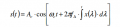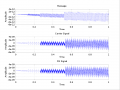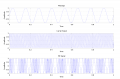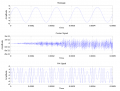# FM Octave Script

#### PsySc0rpi0n

Joined Mar 4, 2014
1,702
Hi everyone...

I'm trying to find a way to display 3 plots with different time bases so that the modulation can be observed!

I mean, I want to modulate a carrier signal by a message signal using FM technique but as the message signal frequency is a few thousand times lower than the carrier frequency, I'm not being able to plot the 3 signals, message, carrier and modulated signals in a way that we can observe the modulation effect.

The signals are:

m (t) = sin (2*π*fm*t) V, where fm = 10kHz

c (t) = sin (2*π*fc*t) V, where fc = 10MHz

The modulated signal is:But I'm using another equation that I suppose to be equivalent to that one!

y = vc*sin(2*pi*fc*t+m.*sin(2*pi*fM*t));

the result is this:I don't like any of the results... Not even the message signal looks like a sin wave... Also the amplitude is weird I don't know why!

If I use lower values, like fm = 8Hz, fc = 100 Hz and modulation index of 10 (same as before) I get a lot better results...Why is this? Why can't I use the script for higher values for frequencies?

clc
clear all
close all

t = 0:0.001:1; %upto 1000 samples

vm = input('Enter Amplitude (Message) = ');
vc = input('Enter Amplitude (Carrier) = ');
fM = input('Enter Message frequency = ');
fC = input('Enter Carrier frequency = ');
m = input('Enter Modulation Index = ');

msg = vm*sin(2*pi*fM*t);
subplot(3,1,1); %plotting message signal
plot(t,msg);
xlabel('Time');
ylabel('Amplitude');
title('Message ');
grid on;

carrier = vc*sin(2*pi*fC*t);
subplot(3,1,2); %plotting carrier signal
plot(t,carrier);
xlabel('Time');
ylabel('Amplitude');
title('Carrier Signal');
grid on;

y = vc*sin(2*pi*fC*t+m.*sin(2*pi*fM*t));
subplot(3,1,3);%plotting FM (Frequency Modulated) signal
plot(t,y);
xlabel('Time');
ylabel('Amplitude');
title('FM Signal');
grid on;

#### sailorjoe

Joined Jun 4, 2013
364
I think you need a smaller time step at the frequencies of interest.
Also noticed you have "m." Instead of "m" in your modulation formula.

#### PsySc0rpi0n

Joined Mar 4, 2014
1,702
I think you need a smaller time step at the frequencies of interest.
Also noticed you have "m." Instead of "m" in your modulation formula.
Hum, I can change the 'm.' to 'm' but I think it changes nothing.

Regarding the time base being smaller, I think I already tried it but then I'll get an error of vector's sizes mismatch...

Can you edit the code and change the time base and then I'll try it? Maybe I tried it the wrong way.

#### sailorjoe

Joined Jun 4, 2013
364
I can't edit your script, but at the top you define your time steps like this:
t = 0:0.001:1
t = 0:0.0001:1 Or t = 0:0.00001:1

#### PsySc0rpi0n

Joined Mar 4, 2014
1,702
I can't edit your script, but at the top you define your time steps like this:
t = 0:0.001:1
t = 0:0.0001:1 Or t = 0:0.00001:1
I meant you to copy my code, edit it and post you version here! But let me try that despite the fact that I think I already tried that but then I had problems of vector's sizes mismatches!

#### sailorjoe

Joined Jun 4, 2013
364
on second thought, you could try this:
t = 0:0.00000001:0.001

this will give you ten cycles of the modulation frequency, which should be more than enough to see the modulation in action. And each carrier frequency cycle will get ten time steps.

#### PsySc0rpi0n

Joined Mar 4, 2014
1,702
on second thought, you could try this:
t = 0:0.00000001:0.001

this will give you ten cycles of the modulation frequency, which should be more than enough to see the modulation in action. And each carrier frequency cycle will get ten time steps.

But I need to have 't' and 'tdisp' with the same number of elements or the error "vector's sizes mismatches"!!!

#### sailorjoe

Joined Jun 4, 2013
364
Ok, but I don't see tdisp in the script. What am I missing?

#### PsySc0rpi0n

Joined Mar 4, 2014
1,702
Ok, this is the best I got so far, but I still don't like at all the Carrier wave form at all, don't know why... That looks like noise and noise only!clc
clear all
close all

vm = input('Enter Amplitude (Message) = ');
vc = input('Enter Amplitude (Carrier) = ');
fM = input('Enter Message frequency = ');
fC = input('Enter Carrier frequency = ');
m = input('Enter Modulation Index = ');

t = [0:5/fC:5/fM]; %upto 1000 samples

msg = vm*sin(2*pi*fM*t);
subplot(3,1,1);
plot(t,msg);%plotting message signal
xlabel('Time');
ylabel('Amplitude');
title('Message ');
grid on;

carrier = vc*sin(2*pi*fC*t);
subplot(3,1,2);
plot(t,carrier); %plotting carrier signal
xlabel('Time');
ylabel('Amplitude');
title('Carrier Signal');
grid on;

y = vc*sin(2*pi*fC*t+m*sin(2*pi*fM*t));
subplot(3,1,3);
plot(t,y); %plotting FM (Frequency Modulated) signal
xlabel('Time');
ylabel('Amplitude');
title('FM Signal');
grid on;

#### PsySc0rpi0n

Joined Mar 4, 2014
1,702
Ok, but I don't see tdisp in the script. What am I missing?

Yes, you're right... That variable is in another script... Because I was trying to use 2 different time bases. One to the math and one other to the plots but that way I was getting errors of vector's sizes mismatches!

Right now I just don't like the carrier wave form!

#### sailorjoe

Joined Jun 4, 2013
364
I'm convinced that what you're seeing is a too slow sampling rate on the carrier signal. The carrier has a period of 10e-6 seconds, or 10 microseconds. Your new script has a time step of only 50 microseconds. So you're only sampling once every five cycles. That's why the plots start out so small. After a number of samples, a bit of rounding error puts the samples at a slightly different place on the carrier wave, so the amplitude goes up a bit. Then after another number of samples it happens again.
So the only way to fix it is to decrease the time step to get perhaps ten samples per carrier wave period, alternatively, scale your frequencies to be lower, like a 10 kHz carrier and a 10 Hz modulation.
I recommend t = [0:1/(10*fC):5/fM]

Last edited:

#### PsySc0rpi0n

Joined Mar 4, 2014
1,702
I'm convinced that what you're seeing is a too slow sampling rate on the carrier signal. The carrier has a period of 10e-6 seconds, or 10 microseconds. Your new script has a time step of only 50 microseconds. So you're only sampling once every five cycles. That's why the plots start out so small. After a number of samples, a bit of rounding error puts the samples at a slightly different place on the carrier wave, so the amplitude goes up a bit. Then after another number of samples it happens again.
So the only way to fix it is to decrease the time step to get perhaps ten samples per carrier wave period, alternatively, scale your frequencies to be lower, like a 10 kHz carrier and a 10 Hz modulation.
I recommend t = [0:1/(10*fC):5/fM]

I think I see the problem, but the weird is that the modulated signal looks good using the same time base!

So, you say to use a different time base for the carrier and the modulated signal, yes? I can try your suggestion! Let me see what does it turns out!

#### sailorjoe

Joined Jun 4, 2013
364
I think you misunderstand. I'm suggesting one time base for everything, but I believe the high speed signal drives the time step requirement. So if you have 10 time steps per each carrier cycle, you'll get 10,000 time steps for each modulation signal cycle, which is more than enough.